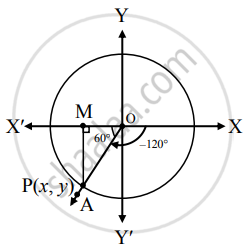# Find the trigonometric functions of : −120° - Mathematics and Statistics

Diagram
Sum

Find the trigonometric functions of :

−120°

#### Solution

Trigonometric Functions of (− 120°) :Let the measure of ∠XOA in Y standard position be - 120°.

Its terminal arm (ray OA) intersects the standard unit circle in P (x, y), which lies in the third quadrant.

Draw seg PM perpendicular to the X-axis.

Then OM = |x| and MP = |y|.

In right-angled triangle OMP,

m∠MOP = 60° and OP = 1

∴ m∠MPO = 30°

∴ OM = 1/2"OP" = 1/2 (1) = 1/2

∴ y2 = PM2  = OP2 - OM2

= 1^2 - (1/2)^2

= 1-1/4

= 3/4

∴ y = ± sqrt(3)/2 and x = ± 1/2

But P lies in the third quadrant.

∴ x < 0 and y < 0

∴ x = -1/2 and y = -sqrt(3)/2

∴ P is (-1/2, -sqrt(3)/2)

∴ sin (– 120°) = y = -sqrt(3)/2

cos (– 120°) = x = -1/2

tan (– 120°) = y/x = ((-(sqrt(3))/2))/((-(1)/2)) = sqrt(3)

cosec (– 120°) = 1/y = 1/((-(sqrt(3))/2)) = -2/sqrt(3)

sec (– 120°) = 1/x = 1/((-(1)/2) = – 2

cot (– 120°) = x/y = ((-(1)/2))/((-sqrt(3)/2)) = 1/sqrt(3)

Concept: Trigonometric Functions of Specific Angles
Is there an error in this question or solution?

#### APPEARS IN

Balbharati Mathematics and Statistics 1 (Arts and Science) 11th Standard Maharashtra State Board
Chapter 2 Trigonometry - 1
Miscellaneous Exercise 2 | Q II. (1) g. | Page 33